# Thom class

Jump to: navigation, search

An element in the (generalized) cohomology group of a Thom space, generating it as a module over the cohomology ring of the base space. For a multiplicative generalized cohomology theory (cf. Generalized cohomology theories)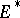, let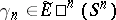be the image of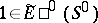under the-fold suspension isomorphism. Letbe an-dimensional vector bundle over a path-connected finite cell complex, and let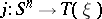be the corresponding inclusion into the Thom space. An element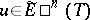is called a Thom class (or orientation) of the bundleif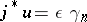, withinvertible in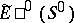. A bundle need not have a Thom class. A bundle with a Thom class (in) is called-orientable, and a bundle with a fixed Thom class is-oriented. The number of Thom classes of an-orientable bundle overis equal to the number of elements of the group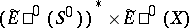. Multiplication by a Thom class gives a Thom isomorphism.

#### Comments

For a (topological) manifold with or without boundary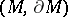, a Thom class is a Thom class for its tangent (micro) bundle. Given a Thom class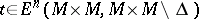, there are isomorphisms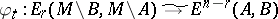(Alexander duality),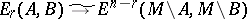,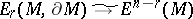(Lefschetz duality) and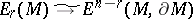,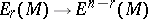(Poincaré duality), whereis a compact triangulable manifold and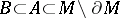are compact subpolyhedra, cf. [a1], Chapt. 14, for more details.

An element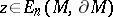is called a fundamental class if for every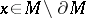one has that(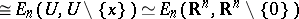) is a generator of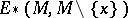as a module over. (Hereis the inclusion.) For the case of ordinary homology, cf. Fundamental class. The relation between a fundamental class and a Thom class is given by the result that ifis a compact triangulable-manifold with Thom class, then there is a unique fundamental classsuch that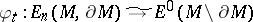takesto, cf. [a1], Prop. 14.17. Using this the Lefschetz and Poincaré duality isomorphisms defined by the Thom class(which essentially are defined by a slant product with) are given by a cap product with.